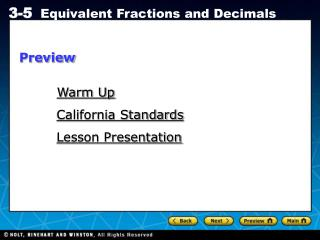Download PresentationWarm Up

# Warm Up - PowerPoint PPT PresentationDownload Presentation## Warm Up

- - - - - - - - - - - - - - - - - - - - - - - - - - - E N D - - - - - - - - - - - - - - - - - - - - - - - - - - -
##### Presentation Transcript

1. Preview Warm Up California Standards Lesson Presentation

2. 4 12 9 23 7 18 25 18 or 1 Warm Up Write each fraction in the simplest form. 1. 2. 3. 4. 18 46 1 3 21 63 1 3 100 72

3. California Standards Preparation for NS1.1 Compare and order positive and negative fractions, decimals, and mixed numbers and place them on a number line. Also covered: NS2.4

4. Vocabulary terminating decimal repeating decimal

5. In baseball, a player’s batting average compares the number of hits with the number of times the player has been at bat. Hits At Bats 172 544 544 172 ÷ 544 ≈ 0.316 172 172 601 172 601 172 ÷ 601 ≈ 0.286 To convert a fraction to a decimal, divide the numerator by the denominator.

6. 1.00 Additional Example 1: Writing Fractions as Decimals Write each fraction as a decimal. Round to the nearest hundredth, if necessary. 9 5 5 3 1 4 B. C. A. 1 .8 1 .6 6 6 0.2 5 9.0 5 5.000 3 4 – 8 – 3 – 5 20 20 40 – 20 – 18 – 40 20 0 0 – 18 9 5 5 3 1 4 = 1.8 ≈ 1.67 = 0.25 20 – 18 2

7. 3.00 Check It Out! Example 1 Write each fraction as a decimal. Round to the nearest hundredth, if necessary. 6 5 7 3 3 4 B. C. A. 1 .2 2 .3 3 3 0.7 5 6.0 5 7.000 3 4 – 2 8 – 6 – 5 20 10 10 – 20 – 9 – 10 10 0 0 – 9 6 5 7 3 3 4 = 1.2 ≈ 2.33 = 0.75 10 – 9 1

8. The decimals 0.75 and 1.2 are terminating decimals because the decimals comes to an end. The decimal 0.333… is a repeating decimal because it includes a group of one or more digits (where all digits are not zero) that repeats forever. You can also write a repeating decimal with a bar over the repeating part. 0.333… = 0.3 0.8333… = 0.83 0.727272… = 0.72

9. 8 10 74 100 Additional Example 2: Using Mental Math to Write Fractions as Decimals Write each fraction as a decimal. 4 5 A. Multiply to get a power of ten in the denominator. 4 5 2 2 = × = 0.8 37 50 B. Multiply to get a power of ten in the denominator. 37 50 2 2 = × = 0.74

10. 6 10 72 100 Check It Out! Example 2 Write each fraction as a decimal. 3 5 A. Multiply to get a power of ten in the denominator. 3 5 2 2 = × = 0.6 18 25 B. Multiply to get a power of ten in the denominator. 18 25 4 4 = × = 0.72

11. You can also use place value to write a terminating decimal as a fraction. Use the place value of the last digit to the right of the decimal point as the denominator of the fraction.

12. Reading Math You read the decimal 0.018 as “eighteen thousandths.” Additional Example 3: Writing Decimals as Fractions Write each decimal as a fraction in simplest form. B. 1.55 A. 0.018 18 1,000 155 100 0.018 = 1.55 = 18 ÷ 2 1,000 ÷ 2 155 ÷ 5 100 ÷ 5 = = 31 20 11 20 9 500 or 1 = =

13. Check It Out! Example 3 Write each decimal as a fraction in simplest form. B. 1.30 A. 0.015 15 1,000 130 100 0.015 = 1.30 = 130 ÷ 10 100 ÷ 10 15 ÷ 5 1,000 ÷ 5 = = 3 200 3 10 13 10 = or 1 =

14. Additional Example 4: Sports Application A football player completed 1,546 of the 3,875 passes he attempted. Find his completion rate. Write your answer as a decimal rounded to the nearest thousandth. 0.3989… Divide the numerator by the denominator. His completion rate is 0.399.

15. Check It Out! Example 4 Johnny Unitas, a former professional quarterback, completed 2,830 of the 5,186 passes he attempted. Find his completion rate. Write your answer as a decimal rounded to the nearest thousandth. 0.5456… Divide the numerator by the denominator. His completion rate is 0.546.

16. Lesson Quiz Write each fraction as a decimal. 1. 3. 4. Write each decimal as a fraction in simplest form. 5. 0.42 7. If your soccer team wins 21 out of 30 games, what is your team’s winning rate? 16 5 21 8 2.625 3.2 2. 7 10 11 20 0.55 0.7 5 8 69 8 21 50 6. 8.625 or 8 0.70